# Constrained triangulation for Simple Features

## Example

This is a basic example which shows you how to decompose a MULTIPOLYGON `sf` data frame object into a GEOMETRYCOLLECTION `sf` data frame object made of triangles:

``library(sf)``
``## Linking to GEOS 3.6.2, GDAL 2.2.4, proj.4 4.9.3``
``````library(sfdct)
nc <- read_sf(system.file("shape/nc.shp", package="sf"), quiet = TRUE)
nc_triangles <- ct_triangulate(nc)

plot(st_geometry(nc_triangles),   col = viridisLite::viridis(nrow(nc_triangles)))``````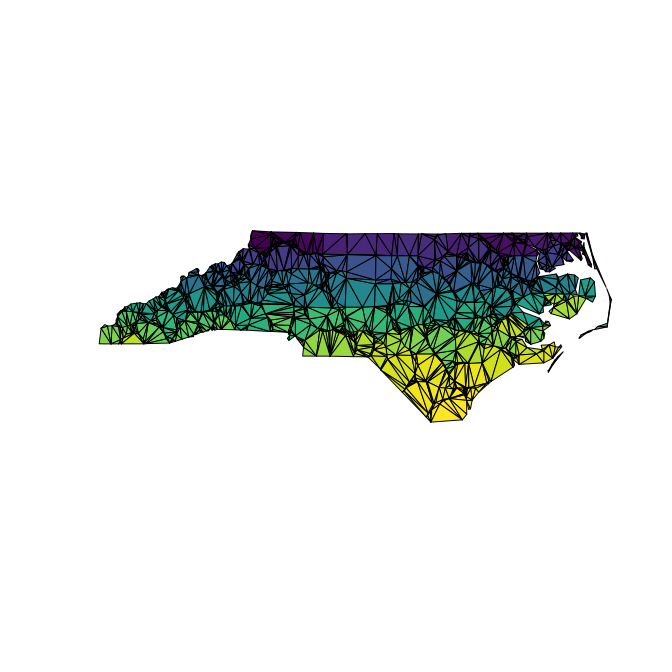We can use the underlying `RTriangle::triangulate` arguments to hone the triangles we get.

``````i_feature <- 25
nc1 <- nc[c(i_feature, unlist(st_touches(nc[i_feature, ], nc))), ]``````
``## although coordinates are longitude/latitude, st_touches assumes that they are planar``
``plot(st_geometry(nc1),col = viridisLite::viridis(nrow(nc1)))``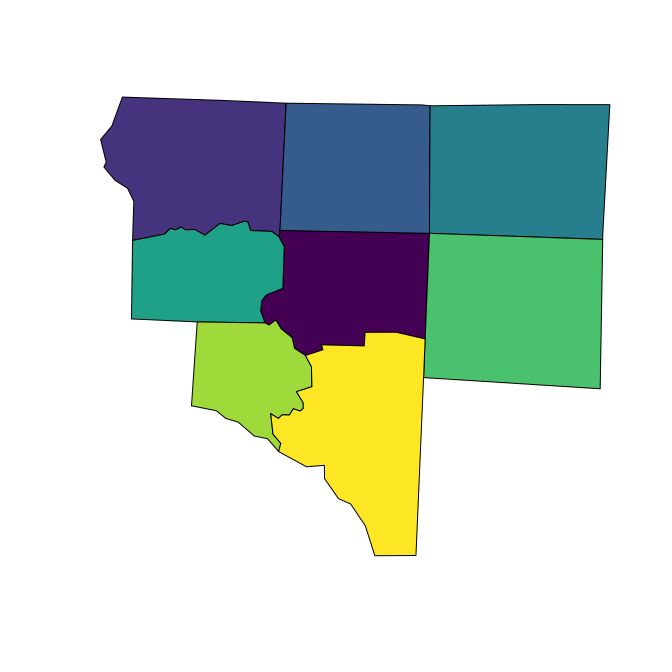``````## subvert st_area because we really don't want m^2
st_crs(nc1) <- NA
areas <- st_area(nc1)
st_crs(nc1) <- st_crs(nc)
nc1_triangles <- ct_triangulate(nc1, a = min(areas)/5)
bcol <- viridisLite::viridis(nrow(nc1_triangles))
plot(st_geometry(nc1_triangles), col = NA, border = bcol)``````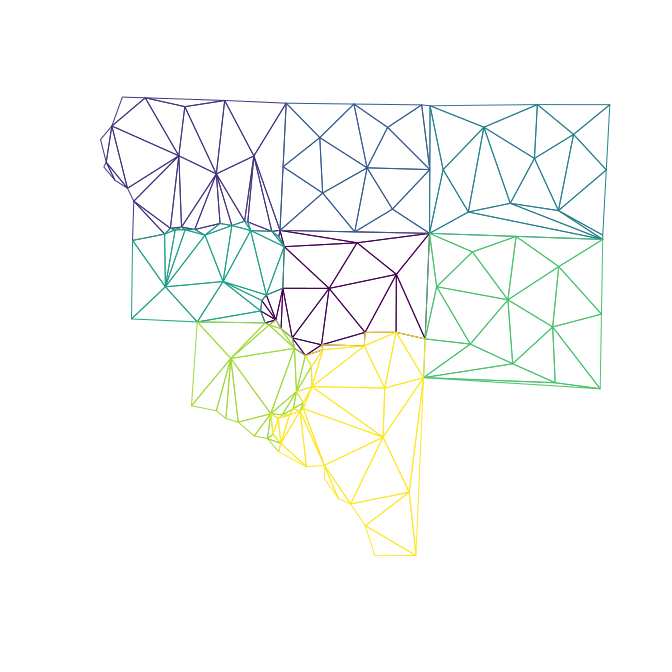``````nc2_triangles <- ct_triangulate(nc1, a = min(st_area(st_set_crs(nc1, NA)))/25)
plot(st_geometry(nc2_triangles), col = NA, border = bcol)``````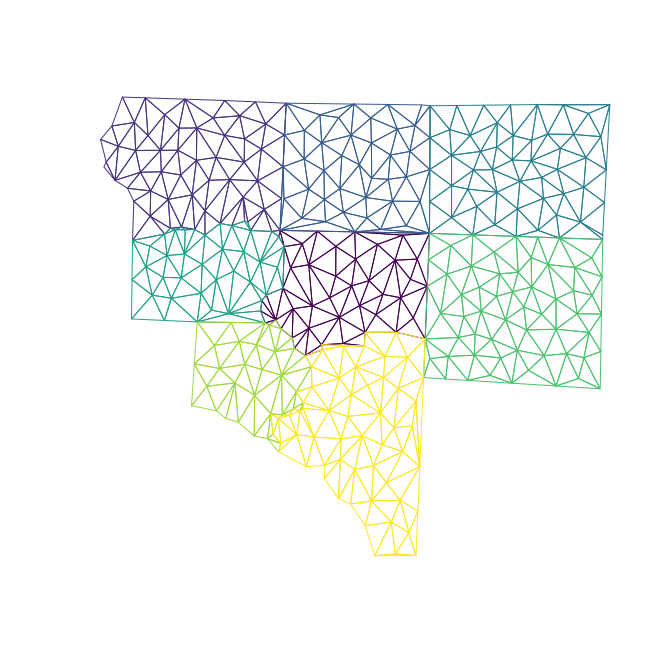Get a grouped triangulated set from a MULTIPOINT. Note how these aren’t constrained by the edges of the input polygons (because we threw those away!) but these are controlled to have a smaller maximum area.

Area is calculated in the native coordinates, assuming “planar coordinates”, with no respect to the real world.

``````## manual cast to MULTIPOINT originally required
#st_geometry(nc1) <- st_sfc(lapply(unlist(unlist(st_geometry(nc1), recursive = FALSE), recursive = FALSE), st_multipoint), crs = st_crs(nc1))
mp_nc1 <- st_cast(nc1, "MULTIPOINT")
mtriangs <- ct_triangulate(nc1, a = 0.0005)
plot(st_geometry(mtriangs), col = viridisLite::viridis(nrow(mtriangs)), border = "#00000033")``````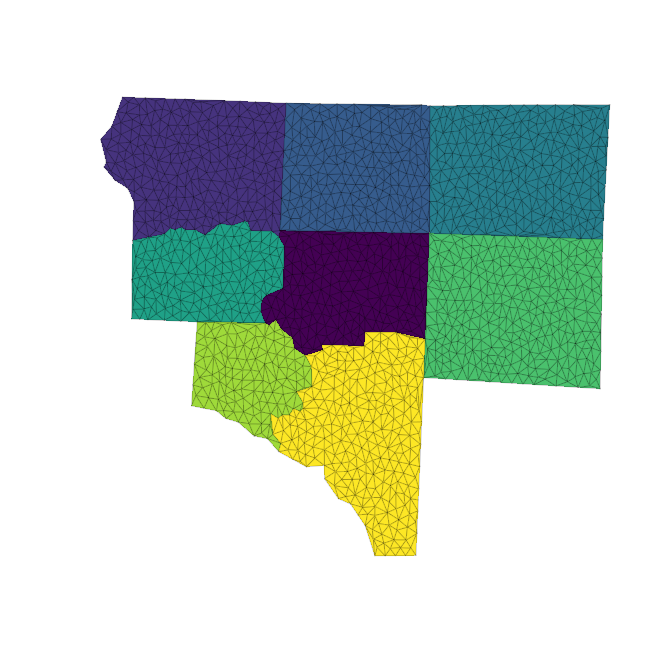``````plot(nc[4, ]\$geometry)
## q, minimum angle
## D, Delaunay criterion is met
plot(ct_triangulate(nc[4, ]\$geometry, q = 35, D = TRUE), add = TRUE, col = "transparent")``````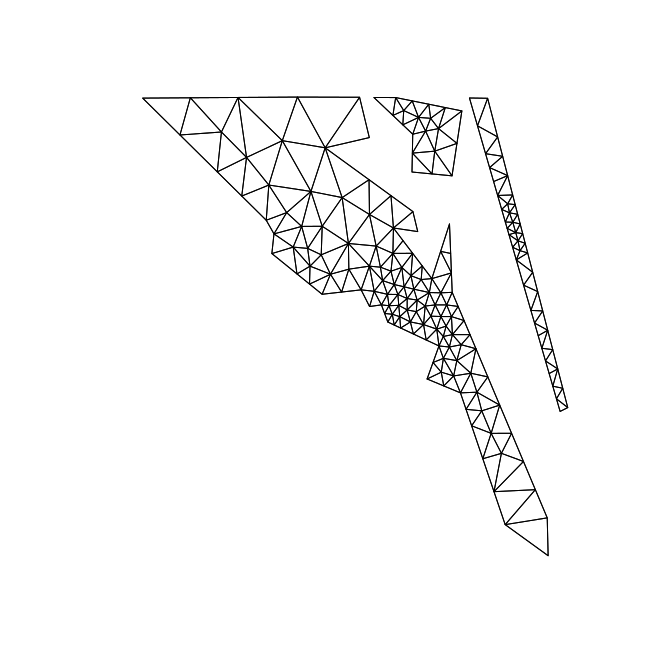## Geometry collection is in development

POLYGON triangles in GEOMETRYCOLLECTION will be re-triangulated. All vertices in the GC will be included, as well as all edges of all component geometries, but each component is triangulated individually, not with reference to the entire set.

## Chaining together operations

We can use piping to chain things together.

``````data("map_world", package= "sfdct")
library(dplyr)``````
``````##
## Attaching package: 'dplyr'``````
``````## The following objects are masked from 'package:stats':
##
##     filter, lag``````
``````## The following objects are masked from 'package:base':
##
##     intersect, setdiff, setequal, union``````
``````g <-  map_world %>% dplyr::filter(startsWith(ID, "Indonesia")) %>% ct_triangulate() %>% st_geometry()
plot(g, col = "aliceblue", main = "")``````